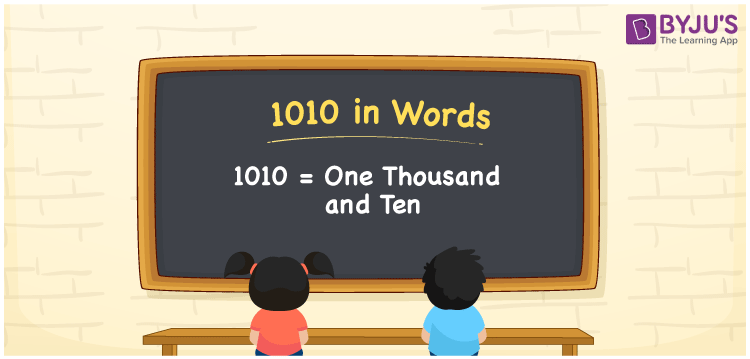# 1010 in Words

We can write 1010 in words as One thousand and ten. We can easily convert the number 1010 into words using a place value chart. If you spent Rs. 1010 in a cafe, you can say that “I spent One thousand and ten rupees at the cafe”. In this article, you will understand how to write the cardinal number 1010 in words.

 1010 in words One thousand and ten One thousand and ten in numerical form 1010

## 1010 in English Words

We generally write numbers in words using the English alphabet. Thus, we can spell 1010 in English as “One thousand and ten”.## How to Write 1010 in Words?

The number 1010 has four digits, so let’s make a place value chart with four columns, as shown below.

 Thousands Hundreds Tens Ones 1 0 1 0

Thus, we can write the expanded form of 1010 using the above place values and digits as:

1 × Thousand + 0 × Hundred + 1 × Ten + 0 × One

= 1 × 1000 + 0 × 100 + 1 × 10 + 0 × 1

= 1000 + 10

= One thousand + Ten

= One thousand and ten

Therefore, 1010 in words = One thousand and ten.

As we know, 1010 is a natural number that precedes 1011 and succeeds 1009.

1010 in words – One thousand and ten

Is 1010 an odd number? – No

Is 1010 an even number? – Yes

Is 1010 a perfect square number? – No

Is 1010 a perfect cube number? – No

Is 1010 a prime number? – No

Is 1010 a composite number? – Yes

## Frequently Asked Questions on 1010 in Words

Q1

### How do you write 1010 in words?

Generally, we write 1010 in English words as One thousand and ten.
Q2

### What is the number name of 1010?

One thousand and ten is the number name of 1010.
Q3

### How to write Rs. 1010 in words for a cheque?

For a cheque, we must write 1010 in words as One thousand and ten rupees only.
Test your Knowledge on 1010 in Words